HistoryMathematiciansAll formulasNum. approx.SoftwaresMisc. math.DigitsPoetryPapers/videosDelirium !Pi-DayImagesMusicLinksBibliography Boris Gourévitch The world of Pi - V2.57 modif. 13/04/2013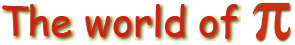HomeVersion historyGuestbookWho I amSome pictures (fr)AcknowledgementsLast modificationsContact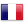Stanley Rabinowitz

The spigot algorithm
A. Sale - D. Saada - S. Rabinowitz

The Principle

So, what is this algorithm?
Well, let's remember that we know pi in its decimal form, which is in base 10:

Pi=3.141592653589793238462643383279...
this can also be written as :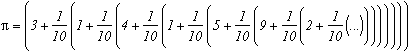This is known as Horner's form; it allows us, among other things, to reduce the number of multiplications when evaluating a polynomial. We can see here that it is base 10, and that the base's step is constant, meaning that we have 10 at each decimal place (digit if base different from 10). We note this base [1/10,1/10,1/10...]
Now, let's consider the series discovered by Euler (See page about him for the demonstration) and let's write it in Horner's form:Now this is interesting, if we compare it with the previous expression, we can see that we are considering a base with a changing step [1,1/3,2/5,3/7,4/9...]. And in this base, Pi is [2;2,2,2,2...]. So in this base, Pi is one of the simpliest numbers that exists !
We know Pi 's digits in this base, so to compute Pi 's decimal places in base 10 one by one, one just needs to build an algorithm that changes it to base 10, which is precisely the principle of the spigot algorithm.

A historical overview of this method

I might as well tell you that I haven't found much information about the above mathematicians! We can't always find what we want on the web, and if someone doesn't have a personal page, it limits what one can find out about him.
Initially, it was A. Sale who had the idea of this method in 1968 and used it to compute e.
Then D. Saada suggested applying it to Pi in 1988 and so did S. Rabinowitz in 1991. The latter is quite well known, he's a confirmed hacker, and even a mathematician who has published quite a few articles (He is a Mac friend by the way). He studied some of the finesses of this algorithm with his collegue Stan Wagon in 1995 in an article in the Mathematical American Monthly.

Around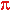:

First of all, a little computation concerning how much memory it will take. In Horner's form, we can see that the n/(2n+1) step of the base is slightly under 1/2 every time. The exact value 1/2 would bring us to consider base 2. For the conversion from base 2 to base 10, we will need roughly Log2(10n)=3,32n digits. We can consider it is about the same value for our base with a changing step to base 10. So if we want to get n décimal places, we will have to consider 3.32n boxes. To get 4 décimal places (including the 3 before the decimal point), we build a memory table of about 14 columns. In fact, 13 will be enough here. The translation of this algorithm would bring us to consider a table like this:

 A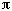r 1 2 3 4 5 6 7 8 9 10 11 12 B = 3 5 7 9 11 13 15 17 19 21 23 25 Initialization 2 2 2 2 2 2 2 2 2 2 2 2 2 *10 20 20 20 20 20 20 20 20 20 20 20 20 20 carried over 10 12 12 12 10 12 7 8 9 0 0 0 0 sum 3 30 32 32 32 30 32 27 28 29 20 20 20 20 remainder 0 2 2 4 3 10 1 13 12 1 20 20 20 *10 0 20 20 40 30 100 10 130 120 10 200 200 200 carried over 13 20 33 40 65 48 98 88 72 150 132 96 0 sum 1 13 40 53 80 95 148 108 218 192 160 332 296 200 remainder 3 1 3 3 5 5 4 8 5 8 17 20 0 *10 30 10 30 30 50 50 40 80 50 80 170 200 0 carried over 11 24 30 40 40 42 63 64 90 120 88 0 0 sum 4 41 34 60 70 90 92 103 144 140 200 258 200 0 remainder 1 1 0 0 0 4 12 9 4 10 6 16 0 *10 10 10 0 0 0 40 120 90 40 100 60 160 0 carried over 4 2 9 24 55 84 63 48 72 60 66 0 0 sum 1 14 12 9 24 55 124 183 138 112 160 126 160 0 remainder 4 0 4 3 1 3 1 3 10 8 0 22 0

And now let's explain how and why it works !
We recognise in the two first lines the numerator and the denominator of the steps of the changing step base. In the third line, we find Pi 's expression in that base. And we fill the last column of the remainder line with 0 's.
The algorithm to convert from right to left in the table is as follows :
Formally, the general principle consists in multiplying the digit by 10 and in computing the remainder in an equivalent of a Euclidian division of this number by the step of the changing step base. A number carried over will then appear at each calculation and comes from the same phenomenon which appears when one multiplies 53 by 8; firstly one multiplies 3 by 8, then one carries over 2 and adds it to the multiplication of 8 by 5 which is the multiplication of the next digit.

Filling in the red column for example :

1) First we fill in the *10 line by multiplying the previous line by 10. No problem so far !
2) We place in sum the sum of the *10 line with the carried over line, which is:

20+9=29
3) We do the Euclidian division of the sum by the number in line B of the same column :

29=17*1+12
4) We place the 12 remainder in the remainder line. Then we multiply the 1 quotient with line A and put the result in the next column's carried over line :

1*8=8
The 9 remainder of the red column comes from the previous column's computation. And so here we pass on the 8 remainder to the next sum's computation.

By repeating the process with the other columns, we fill in the first table and we get 30 as the last sum. But as we multiplied everything by 10, we take 3 as the first digit of Pi. That's it!

One little remark however : We can consider that a number in the last column is right if it is not followed by a 9.
When the remainder in the r column is higher than 100, we can obtain (but it is very rare...) 10 after the last decimal place that we take for Pi. We then take this digit plus 1 as digit for Pi, It's as simple as that!

Other formulas ?

Well I think any rational series should be all right as long as in it's Horner form, we only find integrers as expression of Pi in the changing step base (for the previous series, we only had 2's). Particularly, Ramanujan rational series should be able to be used and give a better algorithm.
Gosper's page's formula is also valid :As the fraction of two terms of the series is always smaller than 1/13, we will need Log13(10n)=0.9 boxes to get a digit. So if we consider 6 boxes, we can hope to get 6*1/0.9=6.6 decimal places, so 6 or even 7 decimal places at best. We can see that this is respected perfectly in the table because we even obtain 7 décimal places :

 Ar 1 6 15 28 45 B = 60 168 330 546 816 Initialization 3 8 13 18 23 28 *10 30 80 130 180 230 280 carried over 1 0 0 0 0 0 sum 3 31 80 130 180 230 280 remainder 1 20 130 180 230 280 *10 10 200 1300 1800 2300 2800 carried over 4 48 75 112 135 0 sum 1 14 248 1375 1912 2435 2800 remainder 4 8 31 262 251 352 *10 40 80 310 2620 2510 3520 carried over 41 12 120 112 180 0 sum 4 41 92 430 2732 2690 3520 remainder 1 32 94 92 506 256 *10 10 320 940 920 5060 2560 carried over 5 30 45 252 135 0 sum 1 15 350 985 1172 5195 2560 remainder 5 50 145 182 281 112 *10 50 500 1450 1820 2810 1120 carried over 9 54 75 140 45 0 sum 5 59 554 1525 1960 2855 1120 remainder 9 14 13 310 125 304 *10 90 140 130 3100 1250 3040 carried over 2 6 135 56 135 0 sum 9 92 146 265 3156 1385 3040 remainder 2 26 97 186 293 592 *10 20 260 970 1860 2930 5920 carried over 4 36 90 140 315 0 sum 2 24 296 1060 2000 3245 5920 remainder 4 56 52 20 515 208